# Rajasthan Board Question Papers for Class 12th Maths 2018 With Solutions In PDF

Rajasthan Class 12 Maths 2018 question paper with solutions are provided here in a downloadable pdf format and also in the text, so that the students get familiar with the pattern and difficulty level of the board exams. Along with the solutions, Maths question paper 2018 Class 12 is also attached here for reference. Students are advised to first solve the RBSE Class 12 Maths Previous Year Question Paper 2018 for practise and then to refer to the solutions, so that they can gauge their preparation level.

Students are able to access all the Rajasthan board previous year Maths question papers. The solutions provided by BYJU’S will assist the students in their preparation and help to score high marks in the upcoming board examination. Instead of solving question papers orally, students should solve each problem individually because most of the time on seeing a question, we feel that we will be able to do it but while writing in the exam we find it difficult to arrive at the solution.

### QUESTION PAPER CODE 1050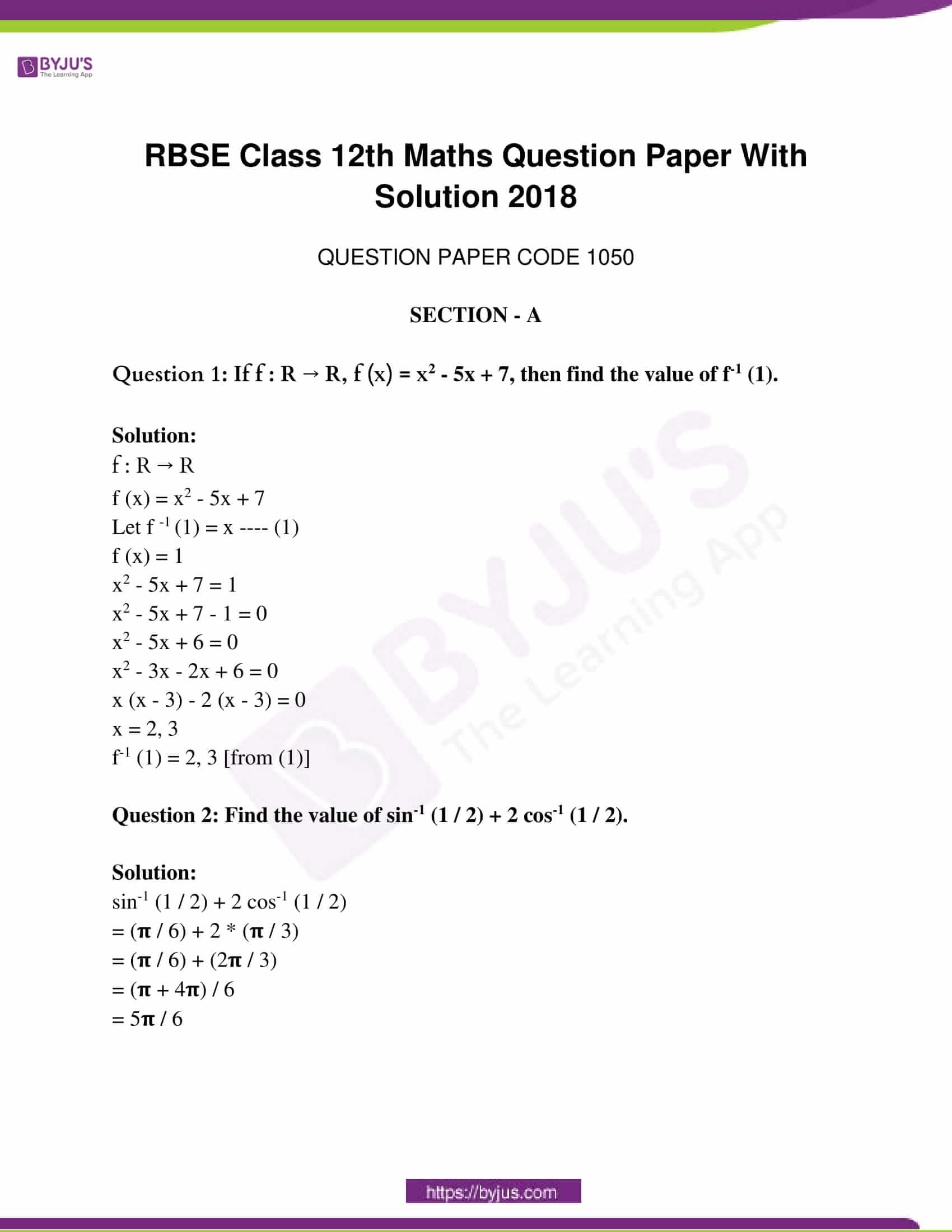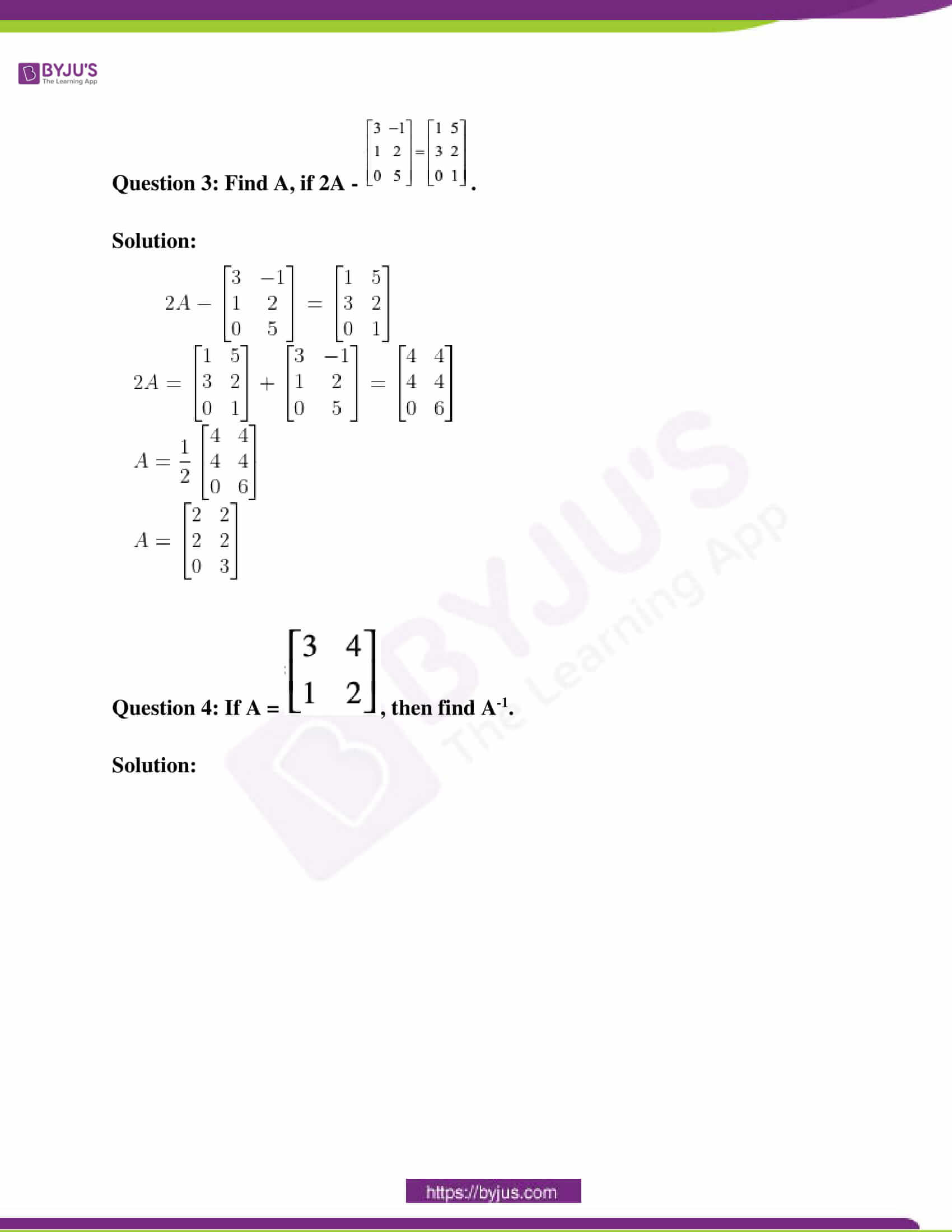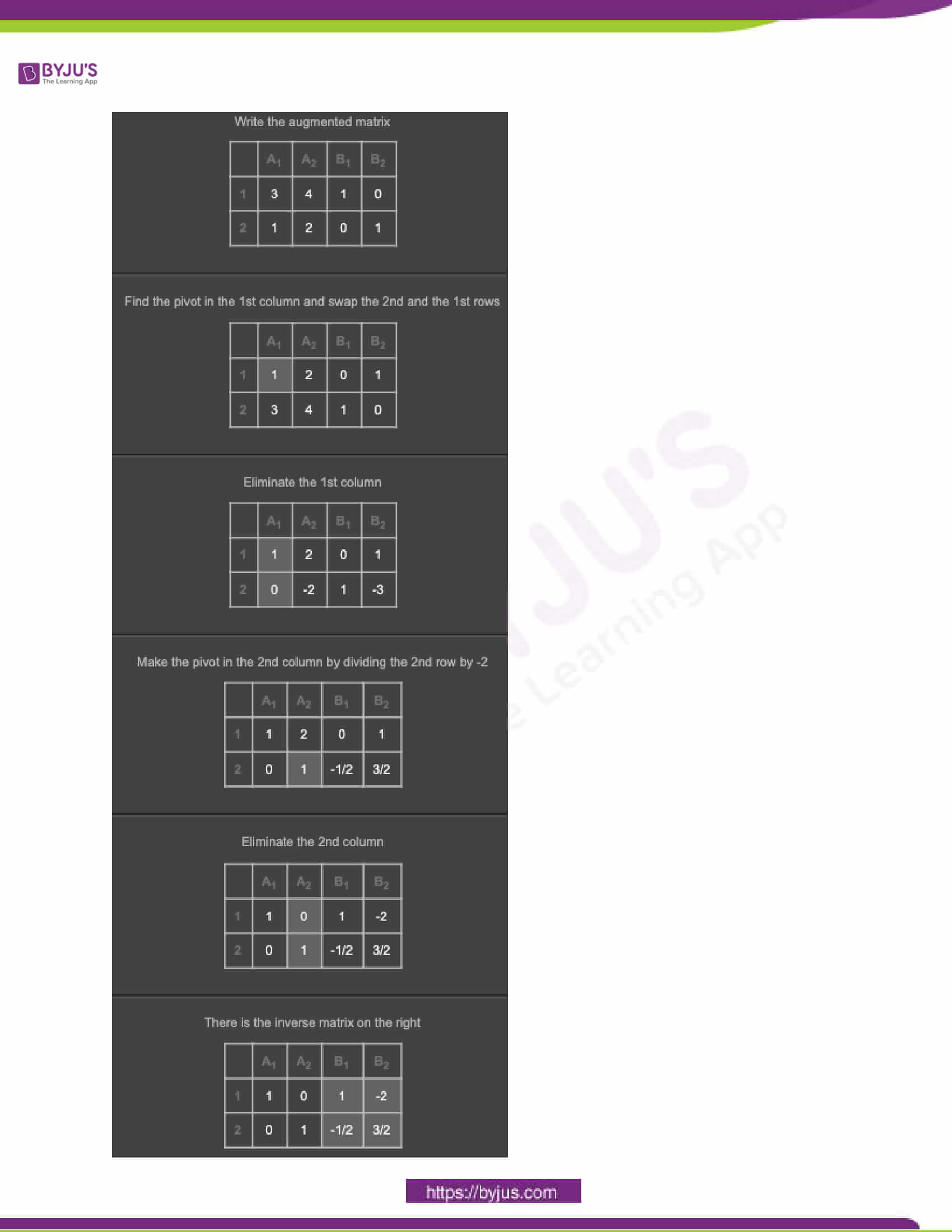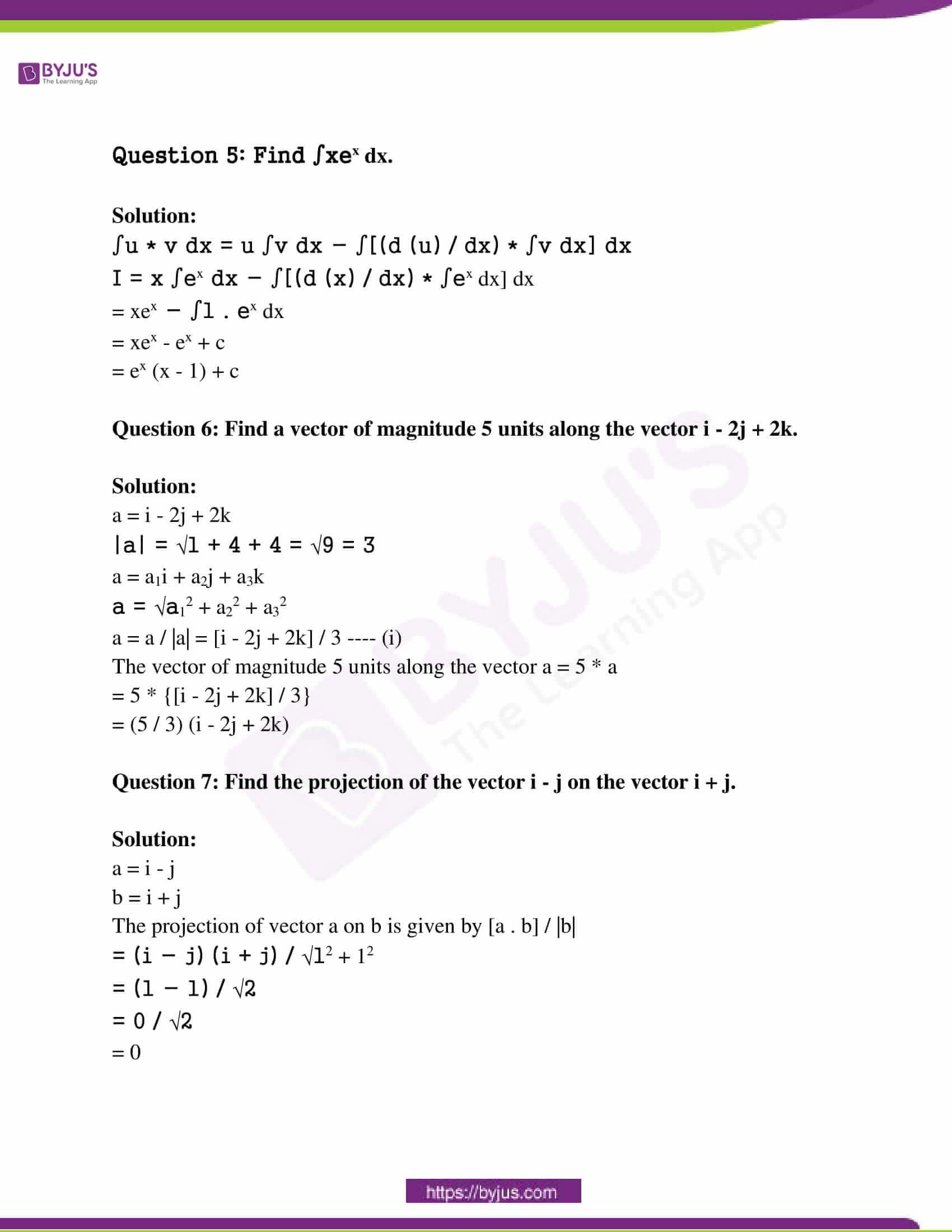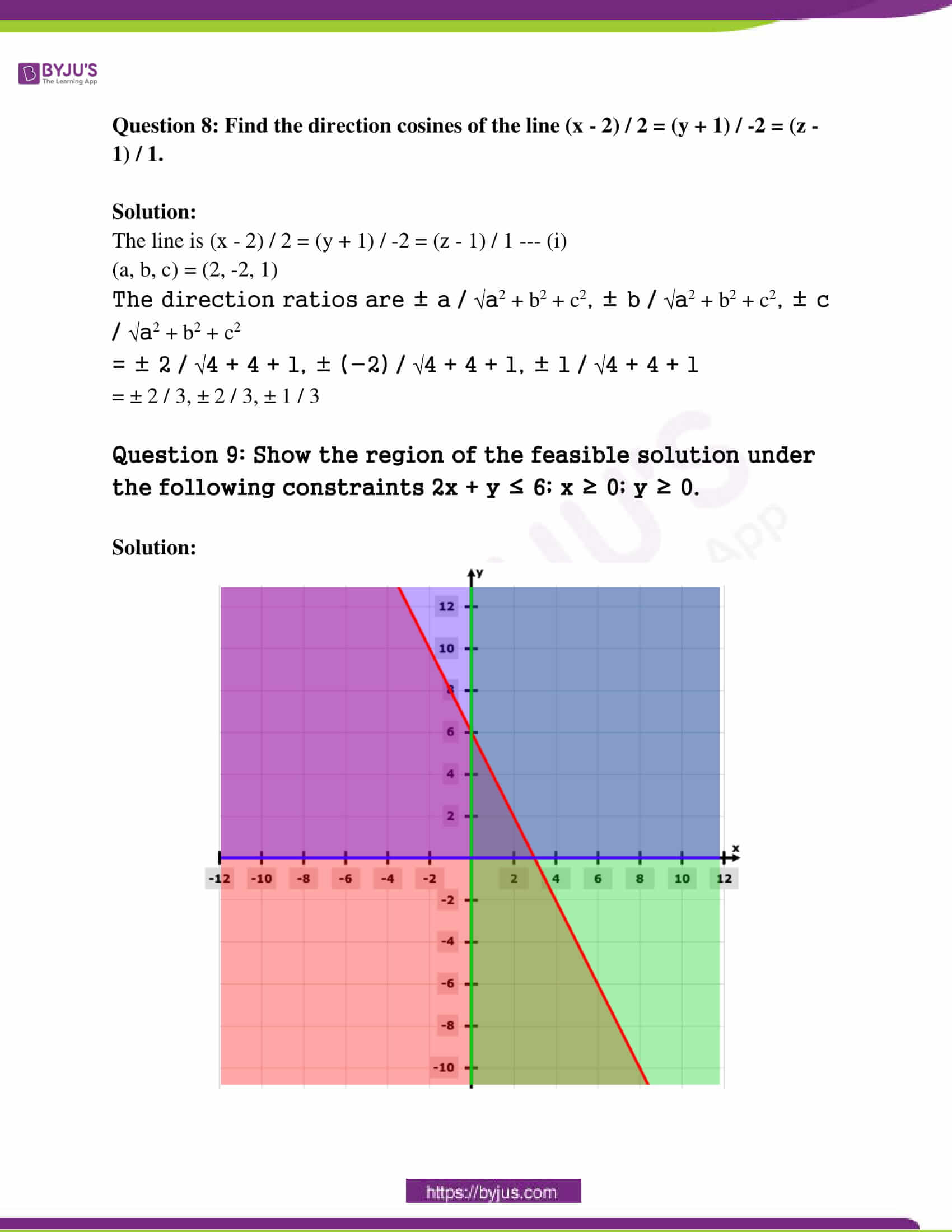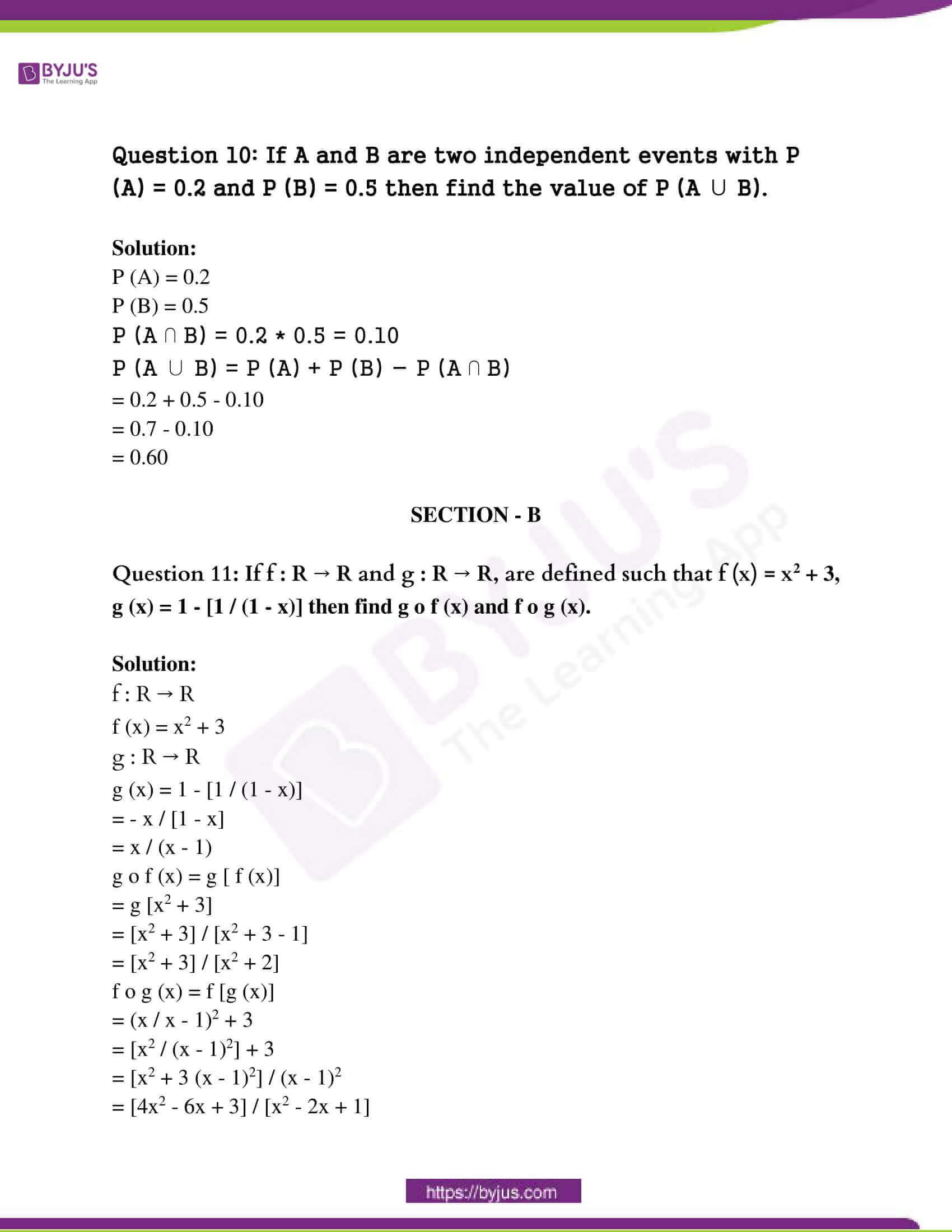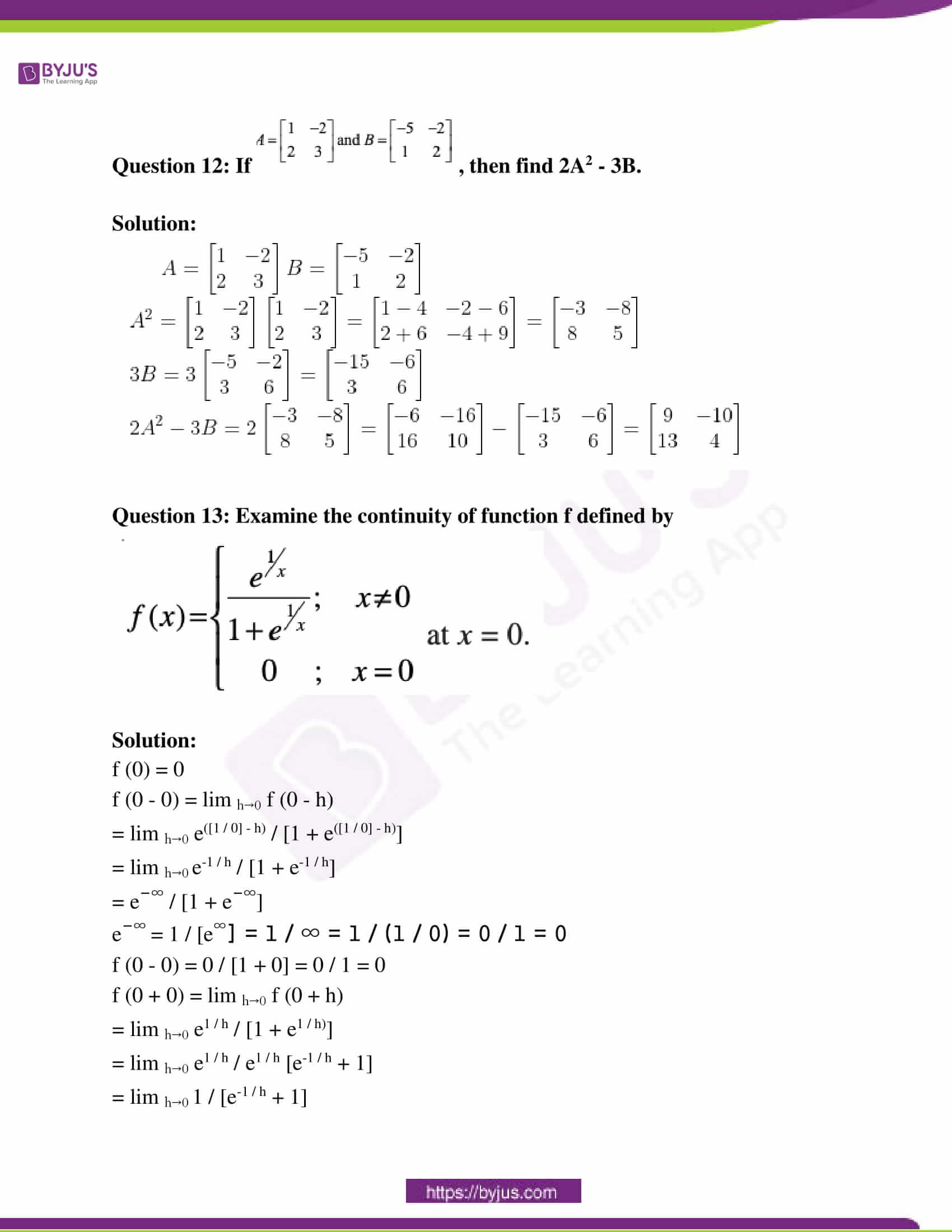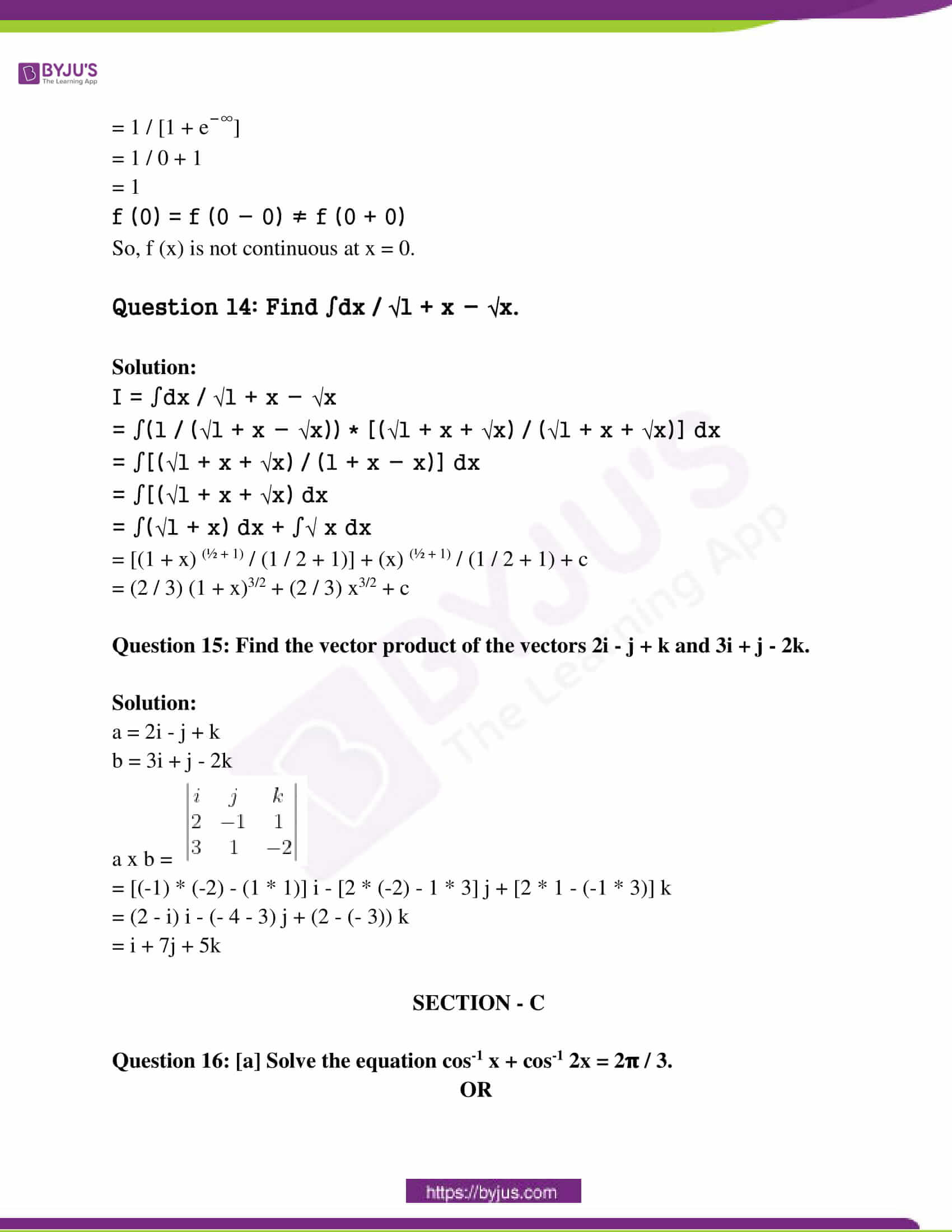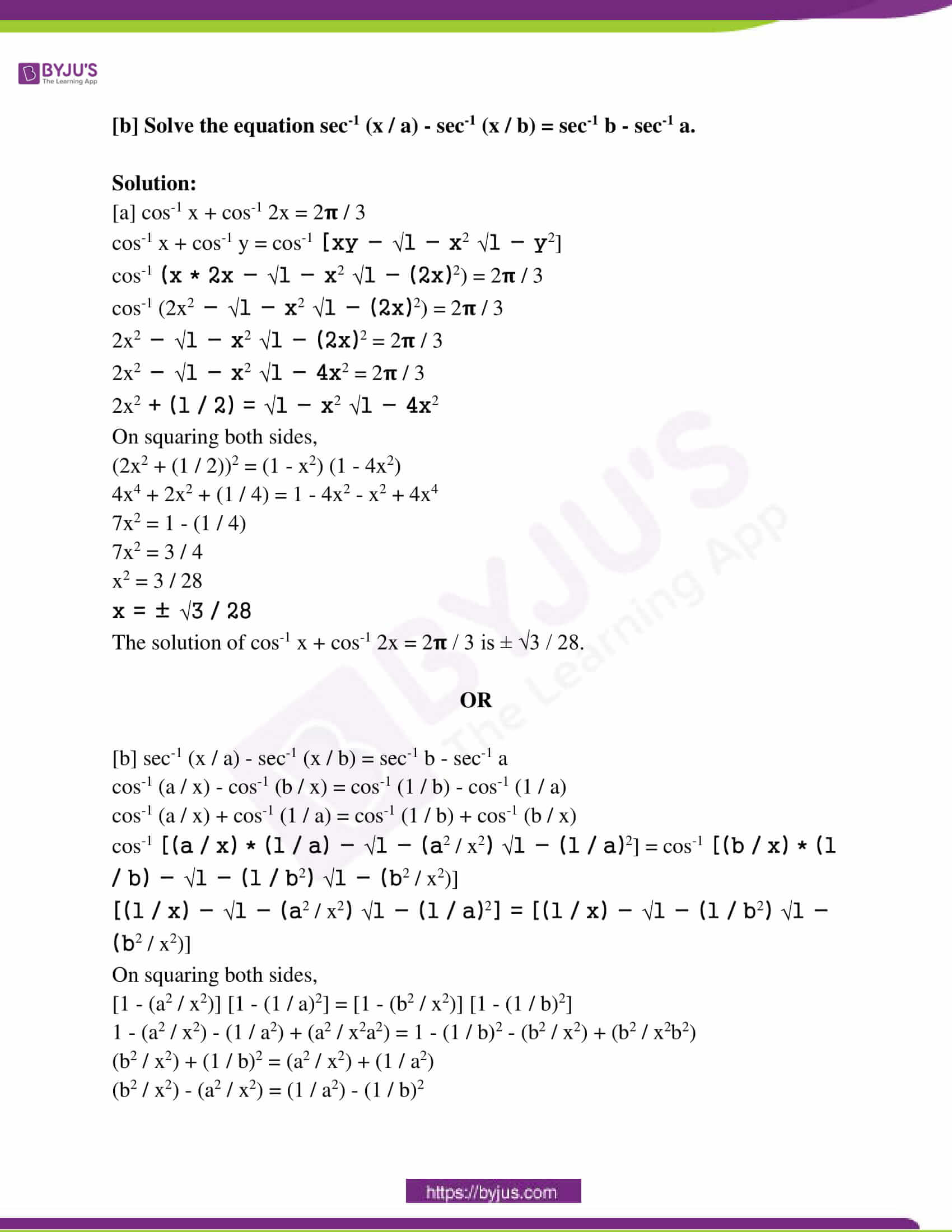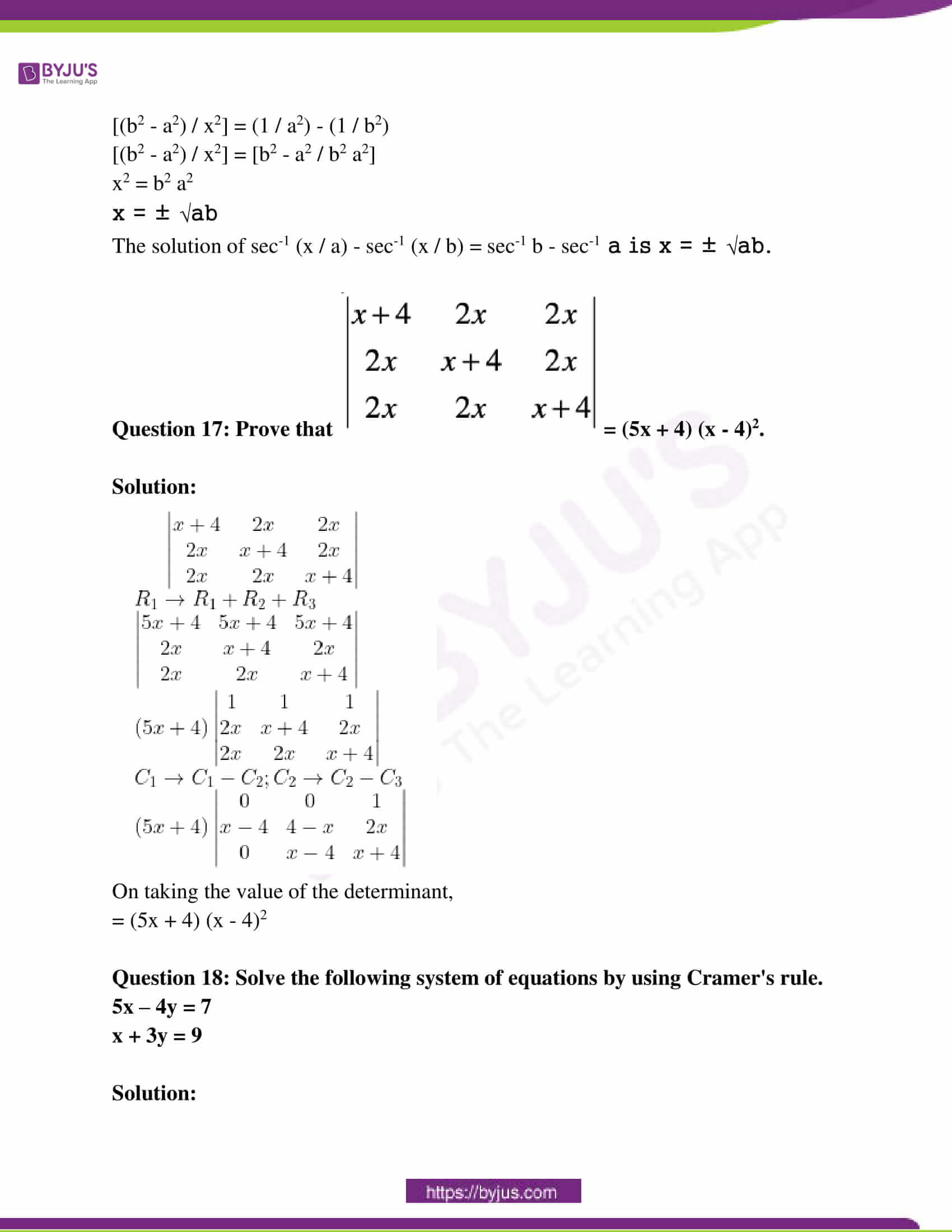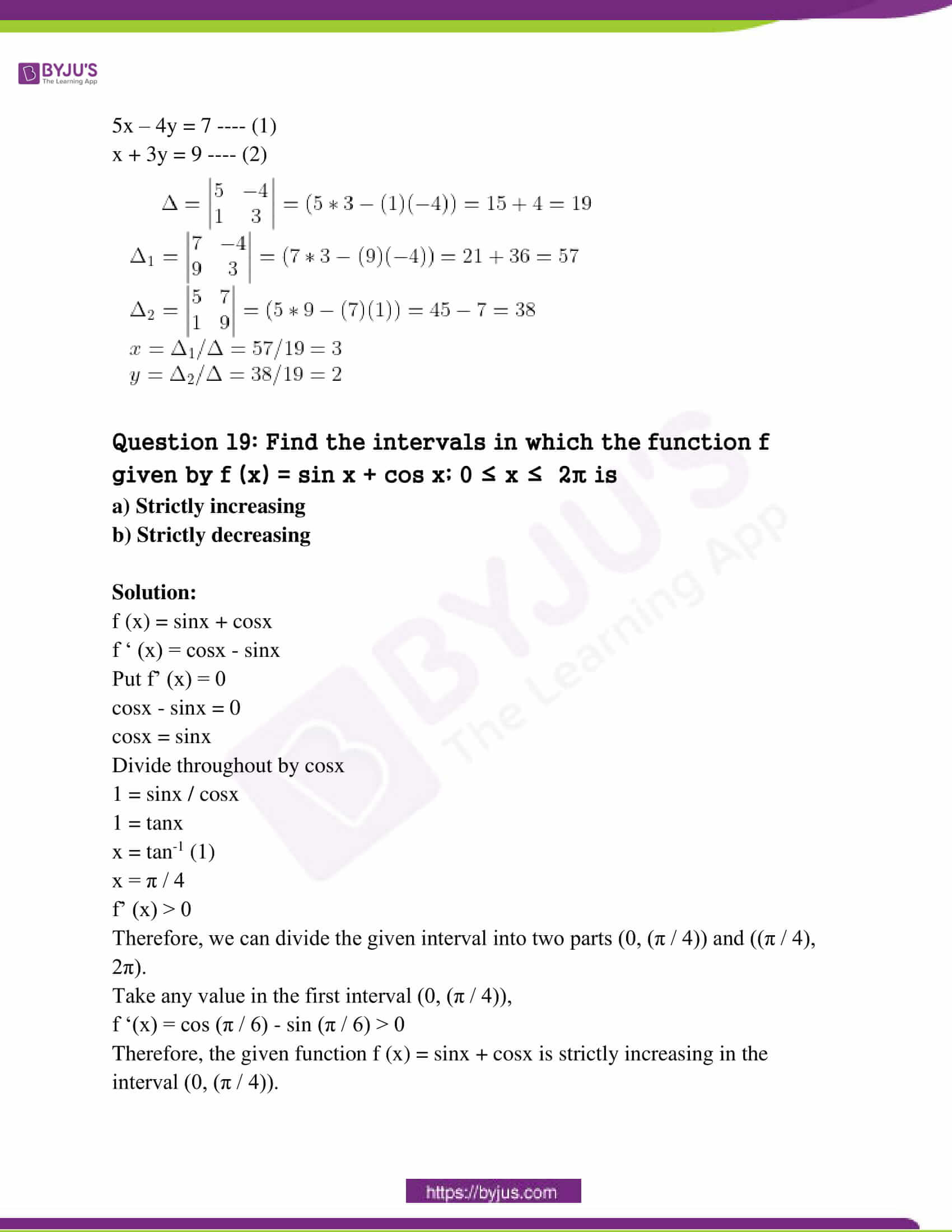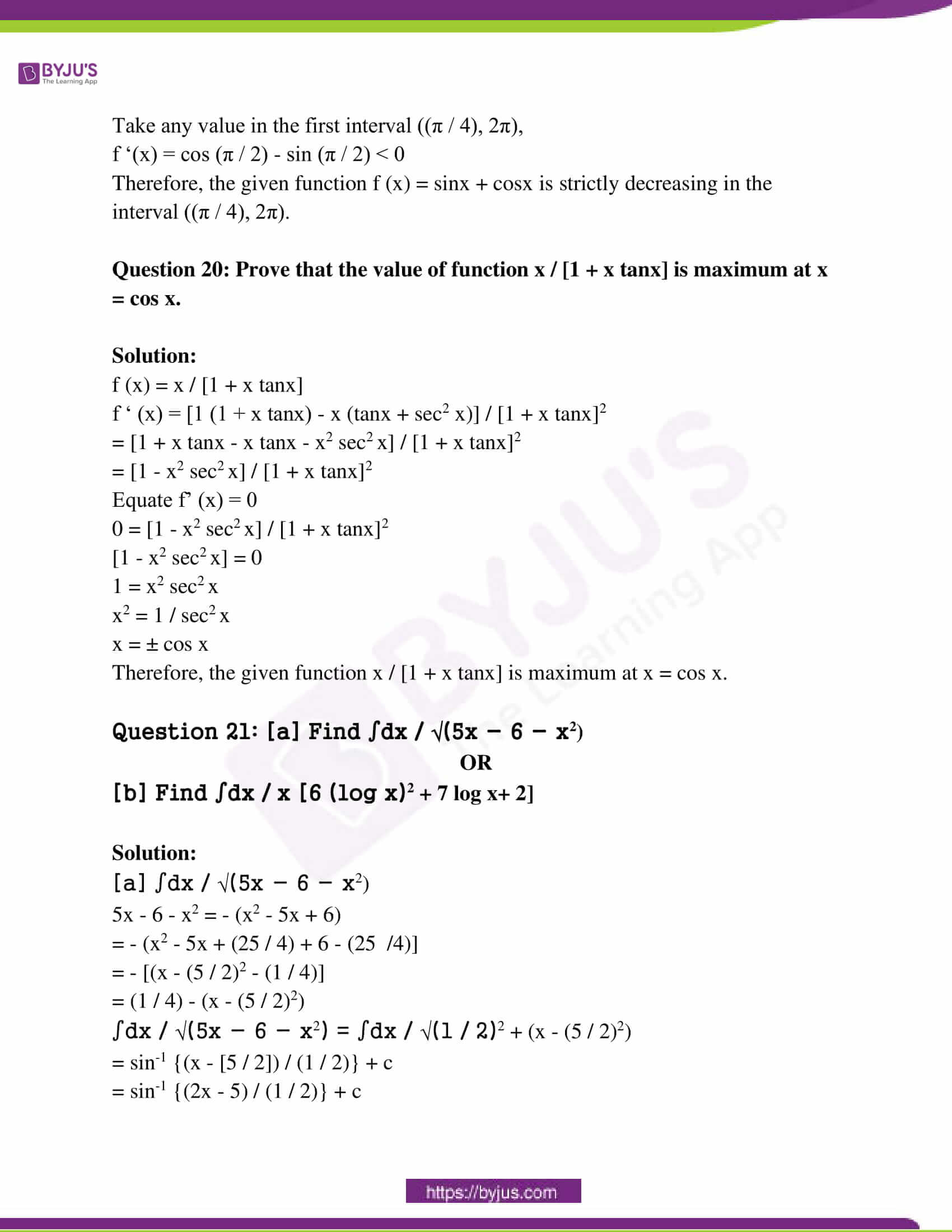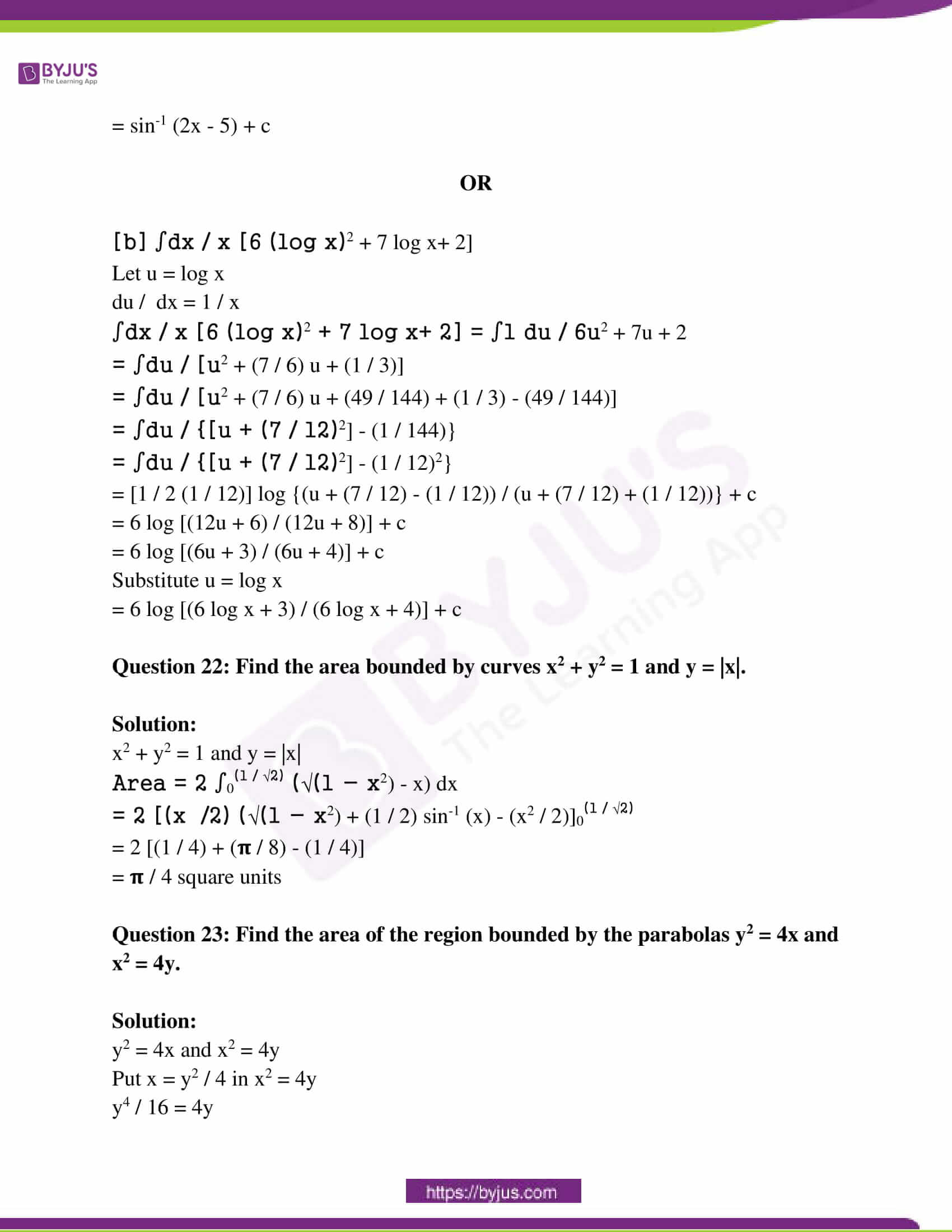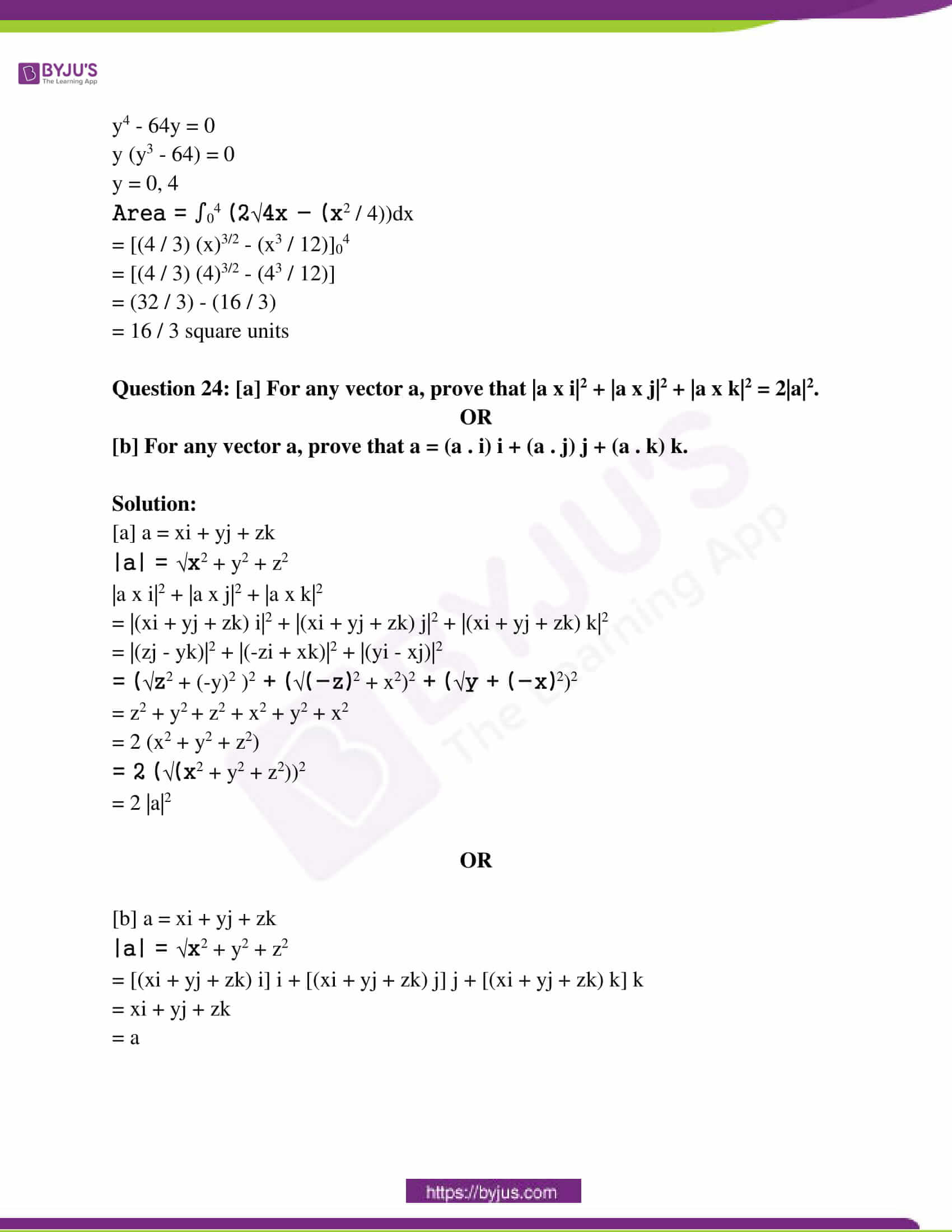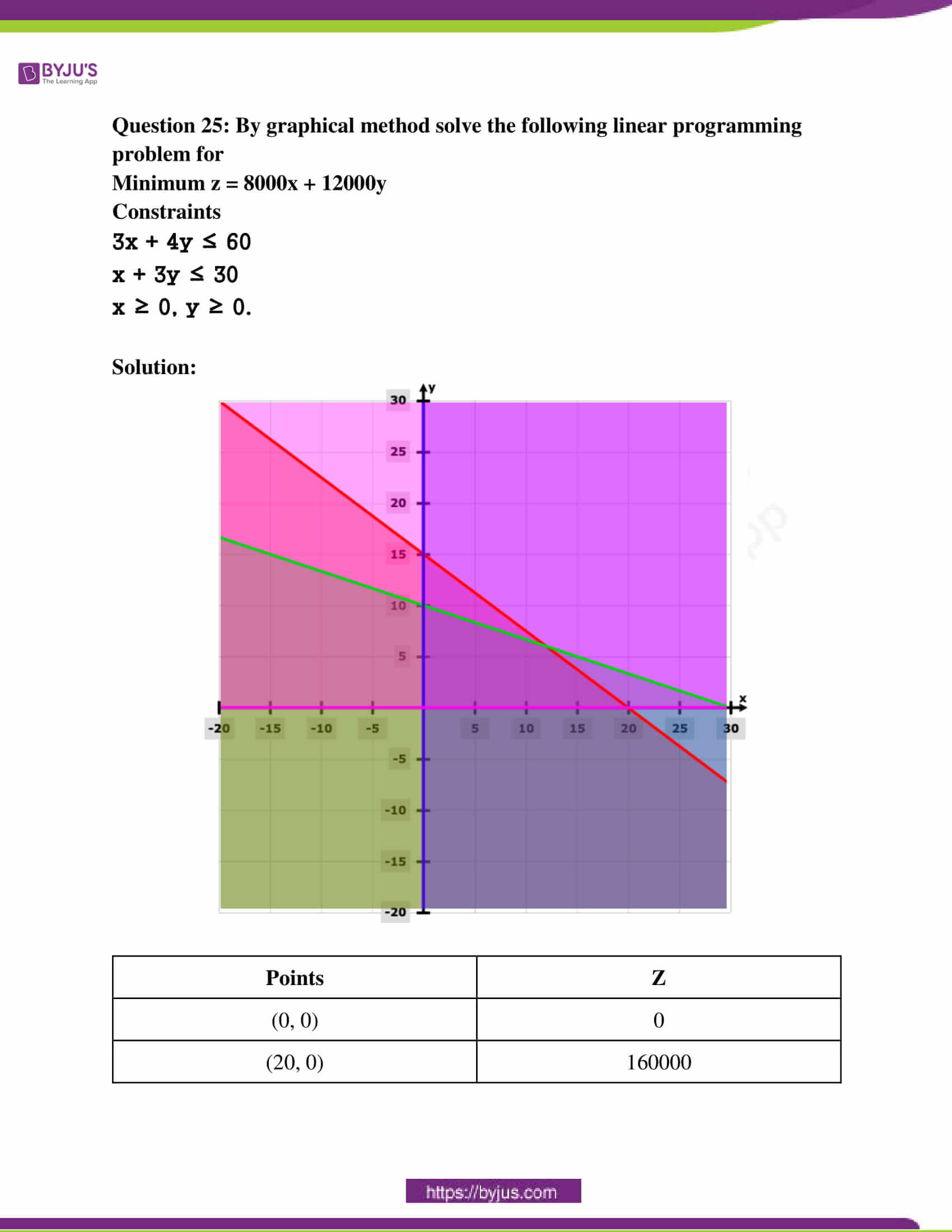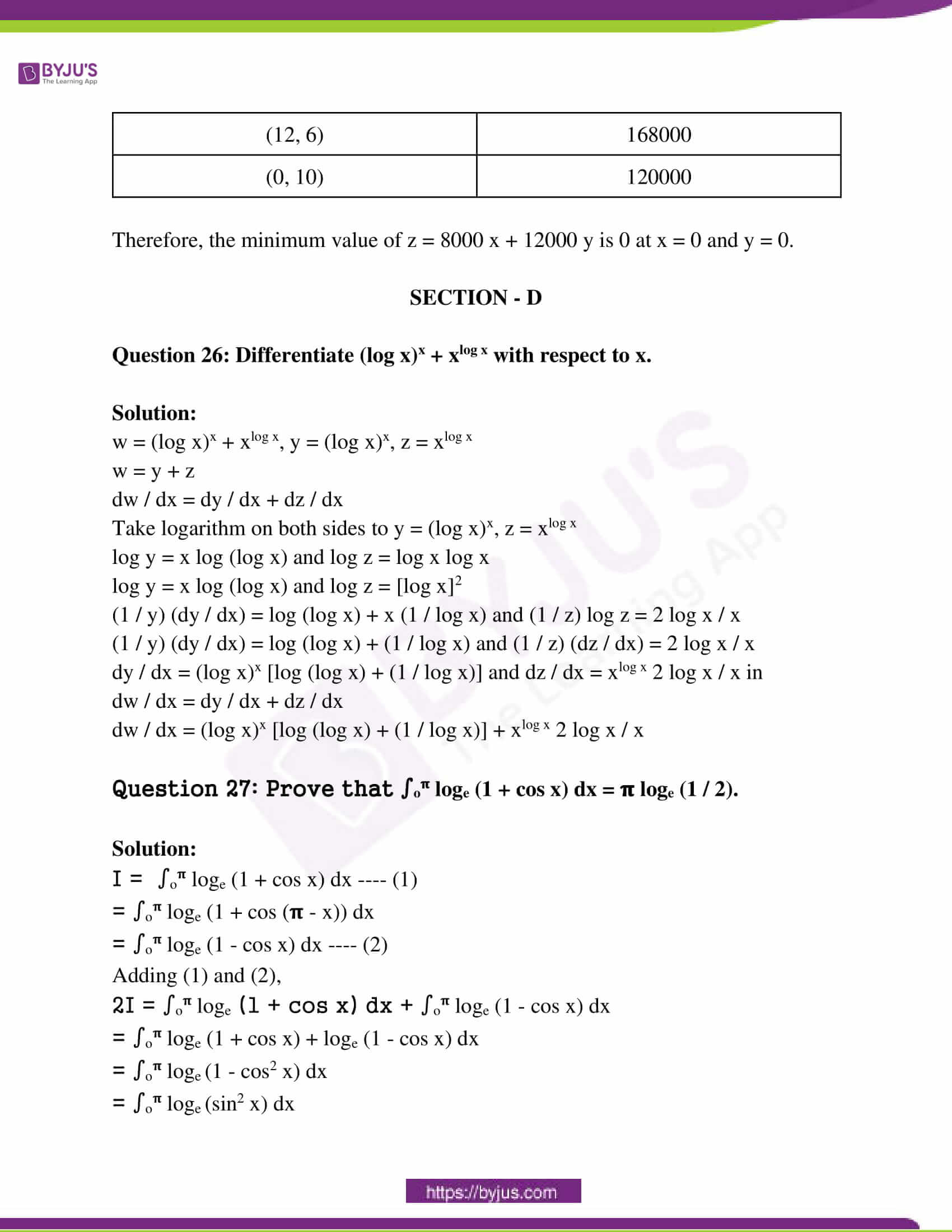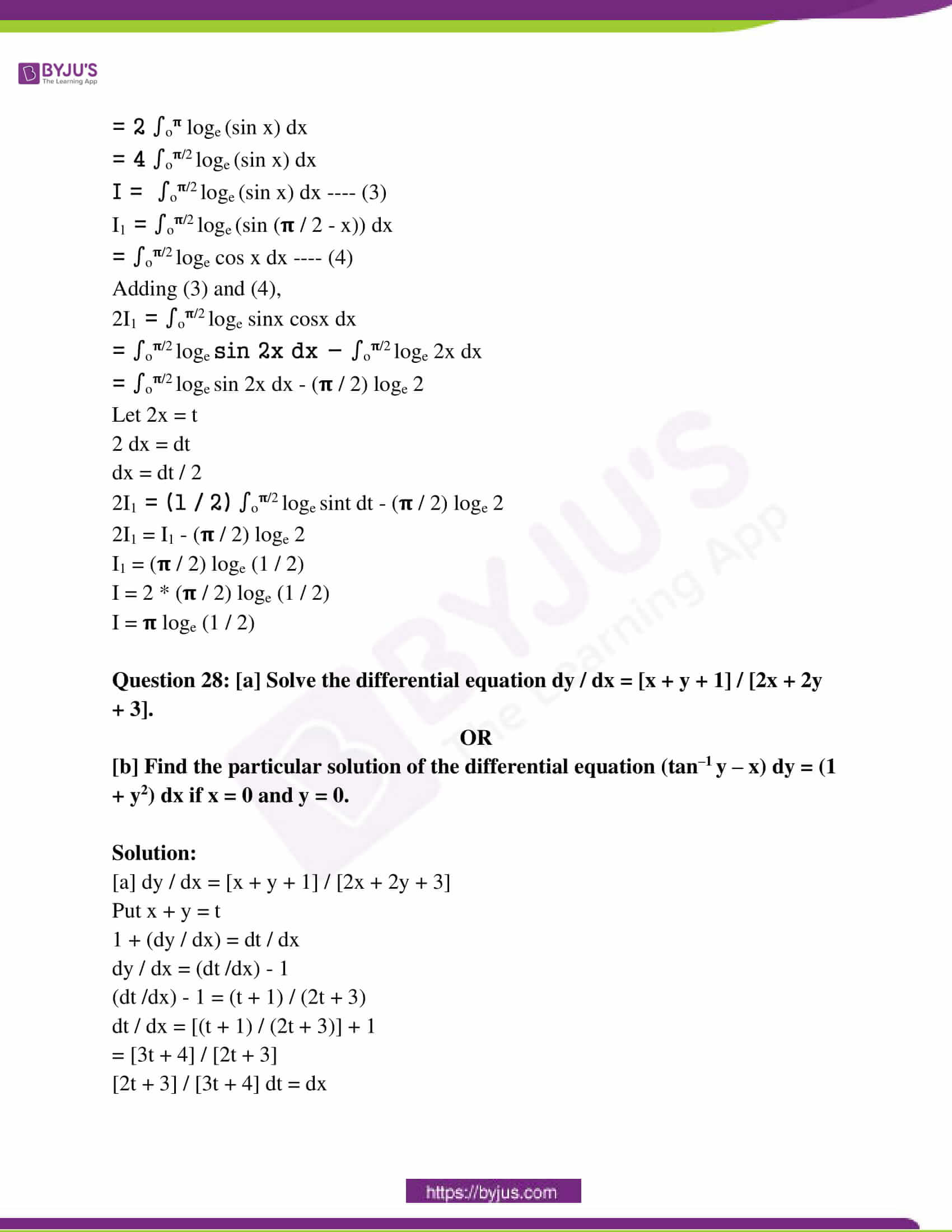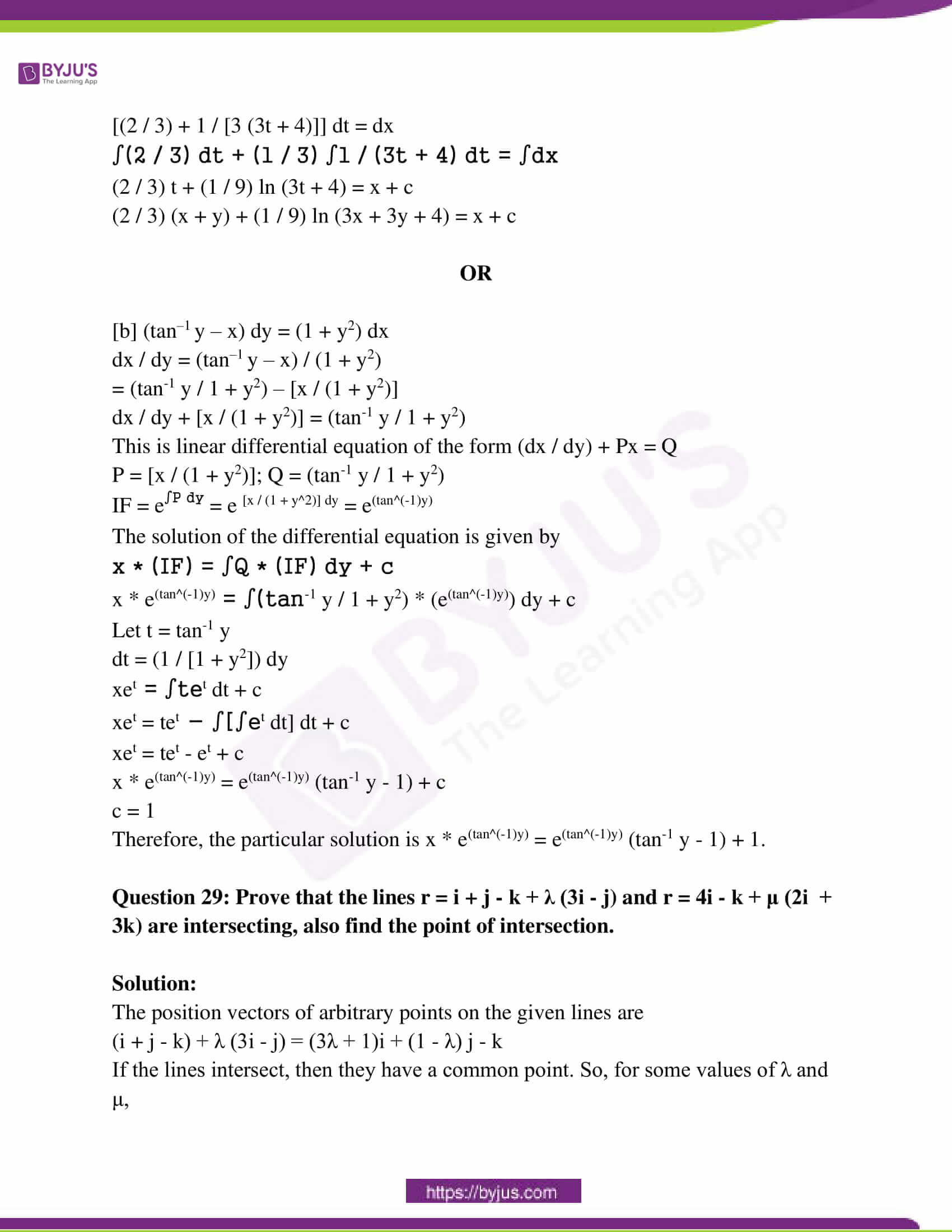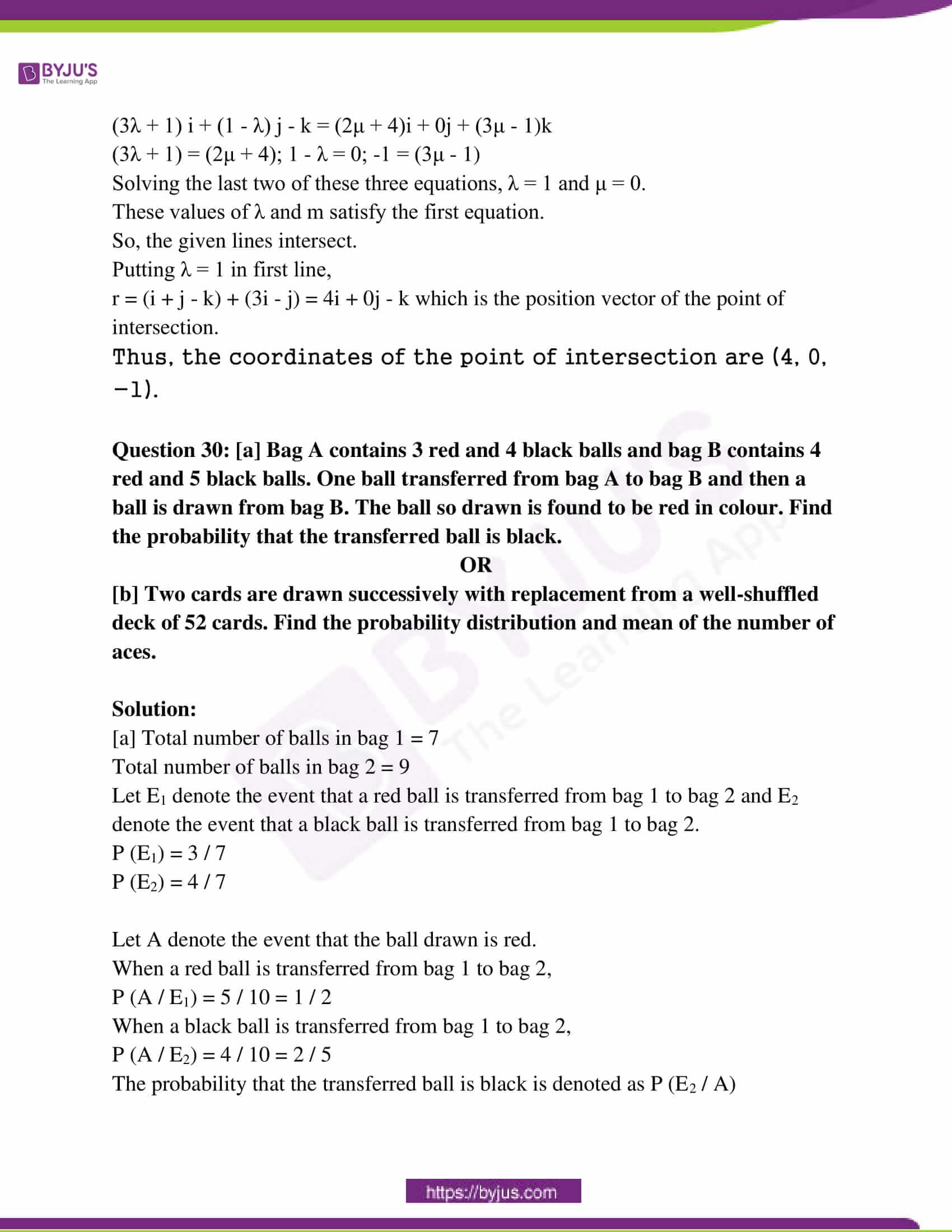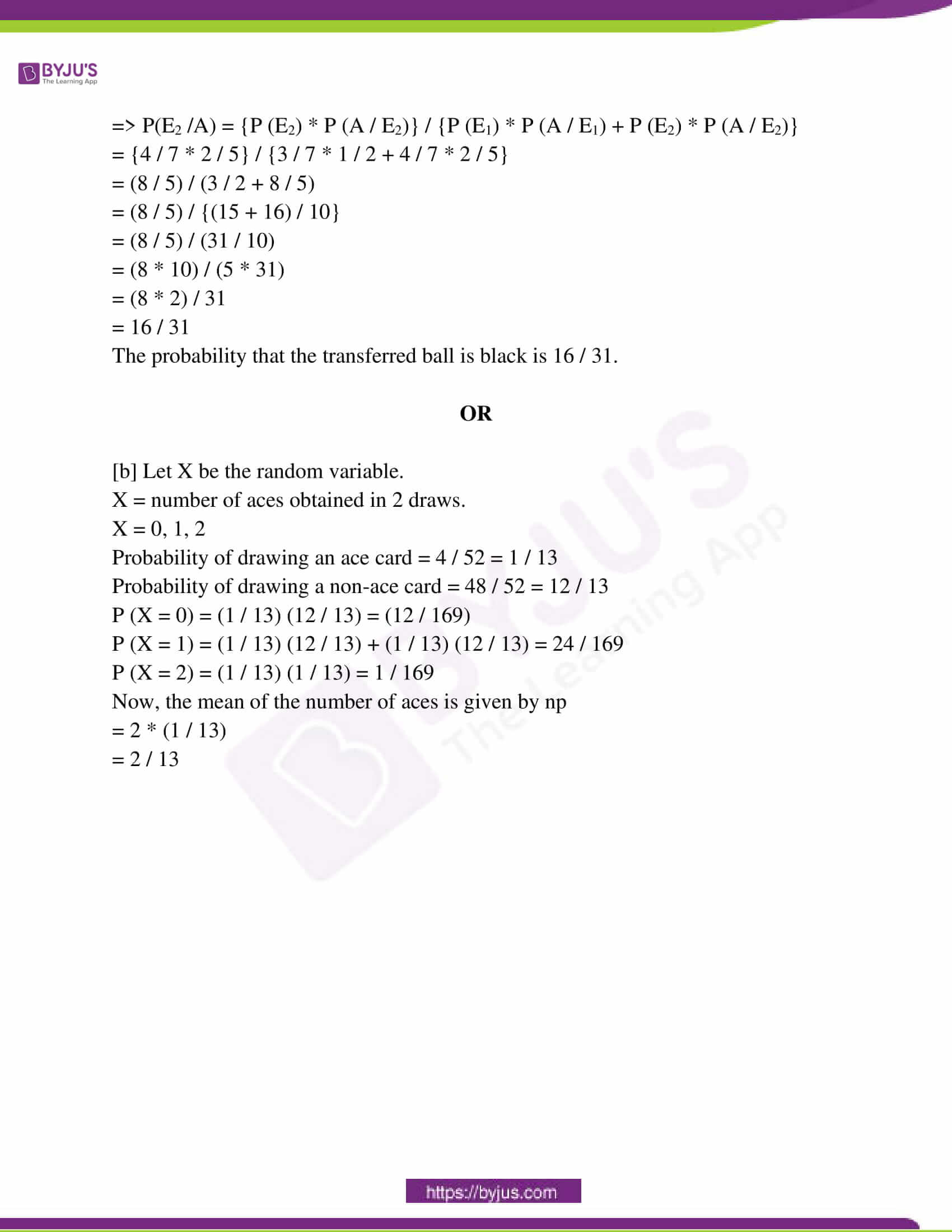SECTION – A

Question 1: If f : R → R, f (x) = x2 – 5x + 7, then find the value of f-1 (1).

Solution:

f : R → R

f (x) = x2 – 5x + 7

Let f -1 (1) = x —- (1)

f (x) = 1

x2 – 5x + 7 = 1

x2 – 5x + 7 – 1 = 0

x2 – 5x + 6 = 0

x2 – 3x – 2x + 6 = 0

x (x – 3) – 2 (x – 3) = 0

x = 2, 3

f-1 (1) = 2, 3 [from (1)]

Question 2: Find the value of sin-1 (1 / 2) + 2 cos-1 (1 / 2).

Solution:

sin-1 (1 / 2) + 2 cos-1 (1 / 2)

= (𝛑 / 6) + 2 * (𝛑 / 3)

= (𝛑 / 6) + (2𝛑 / 3)

= (𝛑 + 4𝛑) / 6

= 5𝛑 / 6

Question 3: Find A, if 2A –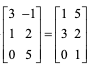.

Solution: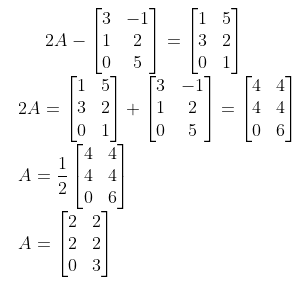Question 4: If A =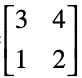, then find A-1.

Solution: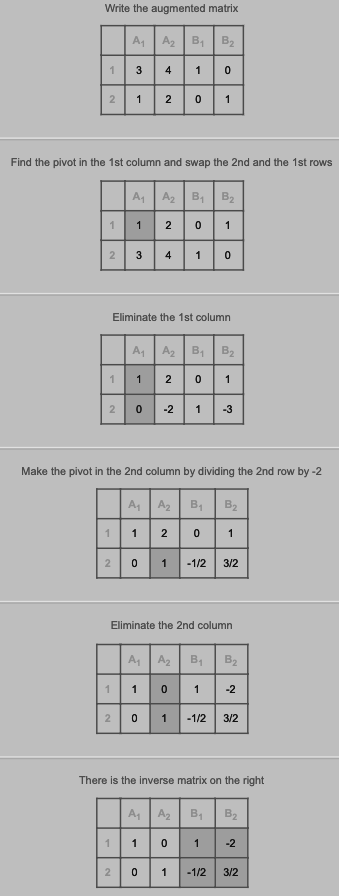Question 5: Find ∫xex dx.

Solution:

∫u * v dx = u ∫v dx – ∫[(d (u) / dx) * ∫v dx] dx

I = x ∫ex dx – ∫[(d (x) / dx) * ∫ex dx] dx

= xex – ∫1 . ex dx

= xex – ex + c

= ex (x – 1) + c

Question 6: Find a vector of magnitude 5 units along the vector i – 2j + 2k.

Solution:

a = i – 2j + 2k

|a| = √1 + 4 + 4 = √9 = 3

a = a1i + a2j + a3k

a = √a12 + a22 + a32

a = a / |a| = [i – 2j + 2k] / 3 —- (i)

The vector of magnitude 5 units along the vector a = 5 * a

= 5 * {[i – 2j + 2k] / 3}

= (5 / 3) (i – 2j + 2k)

Question 7: Find the projection of the vector i – j on the vector i + j.

Solution:

a = i – j

b = i + j

The projection of vector a on b is given by [a . b] / |b|

= (i – j) (i + j) / √12 + 12

= (1 – 1) / √2

= 0 / √2

= 0

Question 8: Find the direction cosines of the line (x – 2) / 2 = (y + 1) / -2 = (z – 1) / 1.

Solution:

The line is (x – 2) / 2 = (y + 1) / -2 = (z – 1) / 1 — (i)

(a, b, c) = (2, -2, 1)

The direction ratios are ± a / √a2 + b2 + c2, ± b / √a2 + b2 + c2, ± c / √a2 + b2 + c2

= ± 2 / √4 + 4 + 1, ± (-2) / √4 + 4 + 1, ± 1 / √4 + 4 + 1

= ± 2 / 3, ± 2 / 3, ± 1 / 3

Question 9: Show the region of the feasible solution under the following constraints 2x + y ≤ 6; x ≥ 0; y ≥ 0.

Solution: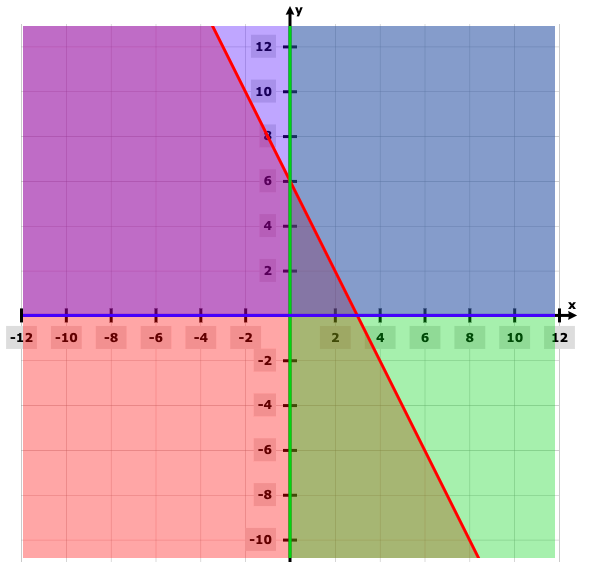Question 10: If A and B are two independent events with P (A) = 0.2 and P (B) = 0.5 then find the value of P (A ∪ B).

Solution:

P (A) = 0.2

P (B) = 0.5

P (A ⋂ B) = 0.2 * 0.5 = 0.10

P (A ∪ B) = P (A) + P (B) – P (A ⋂ B)

= 0.2 + 0.5 – 0.10

= 0.7 – 0.10

= 0.60

SECTION – B

Question 11: If f : R → R and g : R → R, are defined such that f (x) = x2 + 3, g (x) = 1 – [1 / (1 – x)] then find g o f (x) and f o g (x).

Solution:

f : R → R

f (x) = x2 + 3

g : R → R

g (x) = 1 – [1 / (1 – x)]

= – x / [1 – x]

= x / (x – 1)

g o f (x) = g [ f (x)]

= g [x2 + 3]

= [x2 + 3] / [x2 + 3 – 1]

= [x2 + 3] / [x2 + 2]

f o g (x) = f [g (x)]

= (x / x – 1)2 + 3

= [x2 / (x – 1)2] + 3

= [x2 + 3 (x – 1)2] / (x – 1)2

= [4x2 – 6x + 3] / [x2 – 2x + 1]

Question 12: If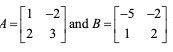, then find 2A2 – 3B.

Solution: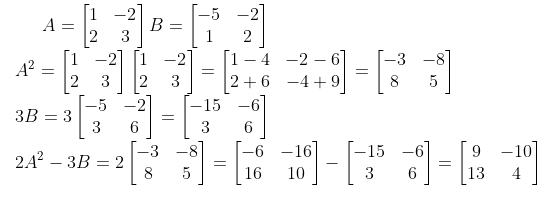Question 13: Examine the continuity of function f defined by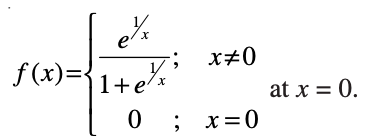Solution:

f (0) = 0

f (0 – 0) = lim h→0 f (0 – h)

= lim h→0 e([1 / 0] – h) / [1 + e([1 / 0] – h)]

= lim h→0 e-1 / h / [1 + e-1 / h]

= e-∞ / [1 + e-∞]

e-∞ = 1 / [e] = 1 / ∞ = 1 / (1 / 0) = 0 / 1 = 0

f (0 – 0) = 0 / [1 + 0] = 0 / 1 = 0

f (0 + 0) = lim h→0 f (0 + h)

= lim h→0 e1 / h / [1 + e1 / h)]

= lim h→0 e1 / h / e1 / h [e-1 / h + 1]

= lim h→0 1 / [e-1 / h + 1]

= 1 / [1 + e-∞]

= 1 / 0 + 1

= 1

f (0) = f (0 – 0) ≠ f (0 + 0)

So, f (x) is not continuous at x = 0.

Question 14: Find ∫dx / √1 + x – √x.

Solution:

I = ∫dx / √1 + x – √x

= ∫(1 / (√1 + x – √x)) * [(√1 + x + √x) / (√1 + x + √x)] dx

= ∫[(√1 + x + √x) / (1 + x – x)] dx

= ∫[(√1 + x + √x) dx

= ∫(√1 + x) dx + ∫√ x dx

= [(1 + x) (½ + 1) / (1 / 2 + 1)] + (x) (½ + 1) / (1 / 2 + 1) + c

= (2 / 3) (1 + x)3/2 + (2 / 3) x3/2 + c

Question 15: Find the vector product of the vectors 2i – j + k and 3i + j – 2k.

Solution:

a = 2i – j + k

b = 3i + j – 2k

a x b =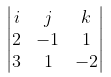= [(-1) * (-2) – (1 * 1)] i – [2 * (-2) – 1 * 3] j + [2 * 1 – (-1 * 3)] k

= (2 – i) i – (- 4 – 3) j + (2 – (- 3)) k

= i + 7j + 5k

SECTION – C

Question 16: [a] Solve the equation cos-1 x + cos-1 2x = 2𝛑 / 3.

OR

[b] Solve the equation sec-1 (x / a) – sec-1 (x / b) = sec-1 b – sec-1 a.

Solution:

[a] cos-1 x + cos-1 2x = 2𝛑 / 3

cos-1 x + cos-1 y = cos-1 [xy – √1 – x2 √1 – y2]

cos-1 (x * 2x – √1 – x2 √1 – (2x)2) = 2𝛑 / 3

cos-1 (2x2 – √1 – x2 √1 – (2x)2) = 2𝛑 / 3

2x2 – √1 – x2 √1 – (2x)2 = 2𝛑 / 3

2x2 – √1 – x2 √1 – 4x2 = 2𝛑 / 3

2x2 + (1 / 2) = √1 – x2 √1 – 4x2

On squaring both sides,

(2x2 + (1 / 2))2 = (1 – x2) (1 – 4x2)

4x4 + 2x2 + (1 / 4) = 1 – 4x2 – x2 + 4x4

7x2 = 1 – (1 / 4)

7x2 = 3 / 4

x2 = 3 / 28

x = ± √3 / 28

The solution of cos-1 x + cos-1 2x = 2𝛑 / 3 is ± √3 / 28.

OR

[b] sec-1 (x / a) – sec-1 (x / b) = sec-1 b – sec-1 a

cos-1 (a / x) – cos-1 (b / x) = cos-1 (1 / b) – cos-1 (1 / a)

cos-1 (a / x) + cos-1 (1 / a) = cos-1 (1 / b) + cos-1 (b / x)

cos-1 [(a / x) * (1 / a) – √1 – (a2 / x2) √1 – (1 / a)2] = cos-1 [(b / x) * (1 / b) – √1 – (1 / b2) √1 – (b2 / x2)] [(1 / x) – √1 – (a2 / x2) √1 – (1 / a)2] = [(1 / x) – √1 – (1 / b2) √1 – (b2 / x2)]

On squaring both sides,

[1 – (a2 / x2)] [1 – (1 / a)2] = [1 – (b2 / x2)] [1 – (1 / b)2]

1 – (a2 / x2) – (1 / a2) + (a2 / x2a2) = 1 – (1 / b)2 – (b2 / x2) + (b2 / x2b2)

(b2 / x2) + (1 / b)2 = (a2 / x2) + (1 / a2)

(b2 / x2) – (a2 / x2) = (1 / a2) – (1 / b)2

[(b2 – a2) / x2] = (1 / a2) – (1 / b2)

[(b2 – a2) / x2] = [b2 – a2 / b2 a2]

x2 = b2 a2

x = ± √ab

The solution of sec-1 (x / a) – sec-1 (x / b) = sec-1 b – sec-1 a is x = ± √ab.

Question 17: Prove that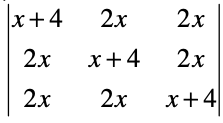= (5x + 4) (x – 4)2.

Solution: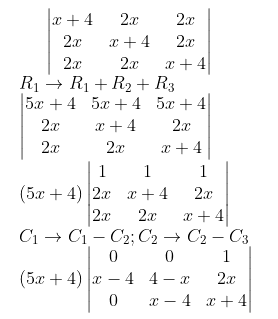On taking the value of the determinant,

= (5x + 4) (x – 4)2

Question 18: Solve the following system of equations by using Cramer’s rule.

5x – 4y = 7

x + 3y = 9

Solution:

5x – 4y = 7 —- (1)

x + 3y = 9 —- (2)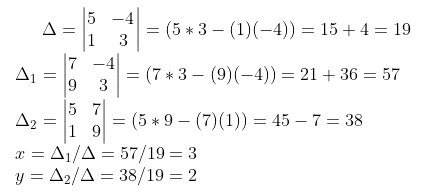Question 19: Find the intervals in which the function f given by f (x) = sin x + cos x; 0 ≤ x ≤ 2π is

a) Strictly increasing

b) Strictly decreasing

Solution:

f (x) = sinx + cosx

f ‘ (x) = cosx – sinx

Put f’ (x) = 0

cosx – sinx = 0

cosx = sinx

Divide throughout by cosx

1 = sinx / cosx

1 = tanx

x = tan-1 (1)

x = π / 4

f’ (x) > 0

Therefore, we can divide the given interval into two parts (0, (π / 4)) and ((π / 4), 2π).

Take any value in the first interval (0, (π / 4)),

f ‘(x) = cos (π / 6) – sin (π / 6) > 0

Therefore, the given function f (x) = sinx + cosx is strictly increasing in the interval (0, (π / 4)).

Take any value in the first interval ((π / 4), 2π),

f ‘(x) = cos (π / 2) – sin (π / 2) < 0

Therefore, the given function f (x) = sinx + cosx is strictly decreasing in the interval ((π / 4), 2π).

Question 20: Prove that the value of function x / [1 + x tanx] is maximum at x = cos x.

Solution:

f (x) = x / [1 + x tanx]

f ‘ (x) = [1 (1 + x tanx) – x (tanx + sec2 x)] / [1 + x tanx]2

= [1 + x tanx – x tanx – x2 sec2 x] / [1 + x tanx]2

= [1 – x2 sec2 x] / [1 + x tanx]2

Equate f’ (x) = 0

0 = [1 – x2 sec2 x] / [1 + x tanx]2

[1 – x2 sec2 x] = 0

1 = x2 sec2 x

x2 = 1 / sec2 x

x = ± cos x

Therefore, the given function x / [1 + x tanx] is maximum at x = cos x.

Question 21: [a] Find ∫dx / √(5x – 6 – x2)

OR

[b] Find ∫dx / x [6 (log x)2 + 7 log x+ 2]

Solution:

[a] ∫dx / √(5x – 6 – x2)

5x – 6 – x2 = – (x2 – 5x + 6)

= – (x2 – 5x + (25 / 4) + 6 – (25 /4)]

= – [(x – (5 / 2)2 – (1 / 4)]

= (1 / 4) – (x – (5 / 2)2)

∫dx / √(5x – 6 – x2) = ∫dx / √(1 / 2)2 + (x – (5 / 2)2)

= sin-1 {(x – [5 / 2]) / (1 / 2)} + c

= sin-1 {(2x – 5) / (1 / 2)} + c

= sin-1 (2x – 5) + c

OR

[b] ∫dx / x [6 (log x)2 + 7 log x+ 2]

Let u = log x

du / dx = 1 / x

∫dx / x [6 (log x)2 + 7 log x+ 2] = ∫1 du / 6u2 + 7u + 2

= ∫du / [u2 + (7 / 6) u + (1 / 3)]

= ∫du / [u2 + (7 / 6) u + (49 / 144) + (1 / 3) – (49 / 144)]

= ∫du / {[u + (7 / 12)2] – (1 / 144)}

= ∫du / {[u + (7 / 12)2] – (1 / 12)2}

= [1 / 2 (1 / 12)] log {(u + (7 / 12) – (1 / 12)) / (u + (7 / 12) + (1 / 12))} + c

= 6 log [(12u + 6) / (12u + 8)] + c

= 6 log [(6u + 3) / (6u + 4)] + c

Substitute u = log x

= 6 log [(6 log x + 3) / (6 log x + 4)] + c

Question 22: Find the area bounded by curves x2 + y2 = 1 and y = |x|.

Solution:

x2 + y2 = 1 and y = |x|

Area = 2 ∫0(1 / √2) (√(1 – x2) – x) dx

= 2 [(x /2) (√(1 – x2) + (1 / 2) sin-1 (x) – (x2 / 2)]0(1 / √2)

= 2 [(1 / 4) + (𝛑 / 8) – (1 / 4)]

= 𝛑 / 4 square units

Question 23: Find the area of the region bounded by the parabolas y2 = 4x and x2 = 4y.

Solution:

y2 = 4x and x2 = 4y

Put x = y2 / 4 in x2 = 4y

y4 / 16 = 4y

y4 – 64y = 0

y (y3 – 64) = 0

y = 0, 4

Area = ∫04 (2√4x – (x2 / 4))dx

= [(4 / 3) (x)3/2 – (x3 / 12)]04

= [(4 / 3) (4)3/2 – (43 / 12)]

= (32 / 3) – (16 / 3)

= 16 / 3 square units

Question 24: [a] For any vector a, prove that |a x i|2 + |a x j|2 + |a x k|2 = 2|a|2.

OR

[b] For any vector a, prove that a = (a . i) i + (a . j) j + (a . k) k.

Solution:

[a] a = xi + yj + zk

|a| = √x2 + y2 + z2

|a x i|2 + |a x j|2 + |a x k|2

= |(xi + yj + zk) i|2 + |(xi + yj + zk) j|2 + |(xi + yj + zk) k|2

= |(zj – yk)|2 + |(-zi + xk)|2 + |(yi – xj)|2

= (√z2 + (-y)2 )2 + (√(-z)2 + x2)2 + (√y + (-x)2)2

= z2 + y2 + z2 + x2 + y2 + x2

= 2 (x2 + y2 + z2)

= 2 (√(x2 + y2 + z2))2

= 2 |a|2

OR

[b] a = xi + yj + zk

|a| = √x2 + y2 + z2

= [(xi + yj + zk) i] i + [(xi + yj + zk) j] j + [(xi + yj + zk) k] k

= xi + yj + zk

= a

Question 25: By graphical method solve the following linear programming problem for

Minimum z = 8000x + 12000y

Constraints

3x + 4y ≤ 60

x + 3y ≤ 30

x ≥ 0, y ≥ 0.

Solution: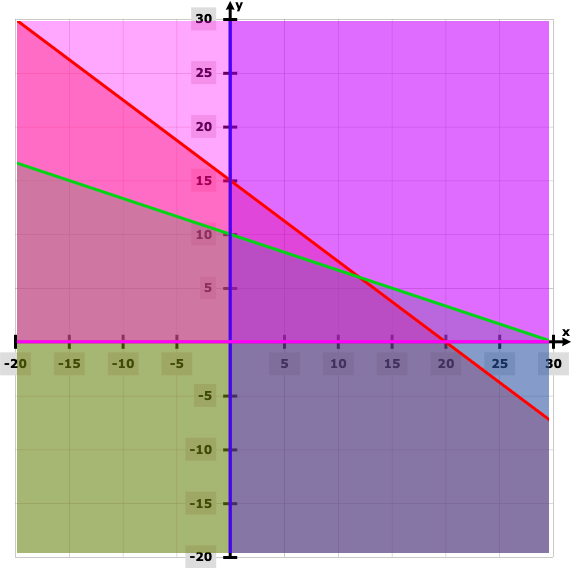Points Z (0, 0) 0 (20, 0) 160000 (12, 6) 168000 (0, 10) 120000

Therefore, the minimum value of z = 8000 x + 12000 y is 0 at x = 0 and y = 0.

SECTION – D

Question 26: Differentiate (log x)x + xlog x with respect to x.

Solution:

w = (log x)x + xlog x, y = (log x)x, z = xlog x

w = y + z

dw / dx = dy / dx + dz / dx

Take logarithm on both sides to y = (log x)x, z = xlog x

log y = x log (log x) and log z = log x log x

log y = x log (log x) and log z = [log x]2

(1 / y) (dy / dx) = log (log x) + x (1 / log x) and (1 / z) log z = 2 log x / x

(1 / y) (dy / dx) = log (log x) + (1 / log x) and (1 / z) (dz / dx) = 2 log x / x

dy / dx = (log x)x [log (log x) + (1 / log x)] and dz / dx = xlog x 2 log x / x in

dw / dx = dy / dx + dz / dx

dw / dx = (log x)x [log (log x) + (1 / log x)] + xlog x 2 log x / x

Question 27: Prove that ∫o𝛑 loge (1 + cos x) dx = 𝛑 loge (1 / 2).

Solution:

I = ∫o𝛑 loge (1 + cos x) dx —- (1)

= ∫o𝛑 loge (1 + cos (𝛑 – x)) dx

= ∫o𝛑 loge (1 – cos x) dx —- (2)

2I = ∫o𝛑 loge (1 + cos x) dx + ∫o𝛑 loge (1 – cos x) dx

= ∫o𝛑 loge (1 + cos x) + loge (1 – cos x) dx

= ∫o𝛑 loge (1 – cos2 x) dx

= ∫o𝛑 loge (sin2 x) dx

= 2 ∫o𝛑 loge (sin x) dx

= 4 ∫o𝛑/2 loge (sin x) dx

I = ∫o𝛑/2 loge (sin x) dx —- (3)

I1 = ∫o𝛑/2 loge (sin (𝛑 / 2 – x)) dx

= ∫o𝛑/2 loge cos x dx —- (4)

2I1 = ∫o𝛑/2 loge sinx cosx dx

= ∫o𝛑/2 loge sin 2x dx – ∫o𝛑/2 loge 2x dx

= ∫o𝛑/2 loge sin 2x dx – (𝛑 / 2) loge 2

Let 2x = t

2 dx = dt

dx = dt / 2

2I1 = (1 / 2) ∫o𝛑/2 loge sint dt – (𝛑 / 2) loge 2

2I1 = I1 – (𝛑 / 2) loge 2

I1 = (𝛑 / 2) loge (1 / 2)

I = 2 * (𝛑 / 2) loge (1 / 2)

I = 𝛑 loge (1 / 2)

Question 28: [a] Solve the differential equation dy / dx = [x + y + 1] / [2x + 2y + 3].

OR

[b] Find the particular solution of the differential equation (tan–1 y – x) dy = (1 + y2) dx if x = 0 and y = 0.

Solution:

[a] dy / dx = [x + y + 1] / [2x + 2y + 3]

Put x + y = t

1 + (dy / dx) = dt / dx

dy / dx = (dt /dx) – 1

(dt /dx) – 1 = (t + 1) / (2t + 3)

dt / dx = [(t + 1) / (2t + 3)] + 1

= [3t + 4] / [2t + 3] [2t + 3] / [3t + 4] dt = dx

[(2 / 3) + 1 / [3 (3t + 4)]] dt = dx

∫(2 / 3) dt + (1 / 3) ∫1 / (3t + 4) dt = ∫dx

(2 / 3) t + (1 / 9) ln (3t + 4) = x + c

(2 / 3) (x + y) + (1 / 9) ln (3x + 3y + 4) = x + c

OR

[b] (tan–1 y – x) dy = (1 + y2) dx

dx / dy = (tan–1 y – x) / (1 + y2)

= (tan-1 y / 1 + y2) – [x / (1 + y2)]

dx / dy + [x / (1 + y2)] = (tan-1 y / 1 + y2)

This is linear differential equation of the form (dx / dy) + Px = Q

P = [x / (1 + y2)]; Q = (tan-1 y / 1 + y2)

IF = e∫P dy = e [x / (1 + y^2)] dy = e(tan^(-1)y)

The solution of the differential equation is given by

x * (IF) = ∫Q * (IF) dy + c

x * e(tan^(-1)y) = ∫(tan-1 y / 1 + y2) * (e(tan^(-1)y)) dy + c

Let t = tan-1 y

dt = (1 / [1 + y2]) dy

xet = ∫tet dt + c

xet = tet – ∫[∫et dt] dt + c

xet = tet – et + c

x * e(tan^(-1)y) = e(tan^(-1)y) (tan-1 y – 1) + c

c = 1

Therefore, the particular solution is x * e(tan^(-1)y) = e(tan^(-1)y) (tan-1 y – 1) + 1.

Question 29: Prove that the lines r = i + j – k + λ (3i – j) and r = 4i – k + μ (2i + 3k) are intersecting, also find the point of intersection.

Solution:

The position vectors of arbitrary points on the given lines are

(i + j – k) + λ (3i – j) = (3λ + 1)i + (1 – λ) j – k

If the lines intersect, then they have a common point. So, for some values of λ and μ,

(3λ + 1) i + (1 – λ) j – k = (2μ + 4)i + 0j + (3μ – 1)k

(3λ + 1) = (2μ + 4); 1 – λ = 0; -1 = (3μ – 1)

Solving the last two of these three equations, λ = 1 and μ = 0.

These values of λ and m satisfy the first equation.

So, the given lines intersect.

Putting λ = 1 in first line,

r = (i + j – k) + (3i – j) = 4i + 0j – k which is the position vector of the point of intersection.

Thus, the coordinates of the point of intersection are (4, 0, −1).

Question 30: [a] Bag A contains 3 red and 4 black balls and bag B contains 4 red and 5 black balls. One ball transferred from bag A to bag B and then a ball is drawn from bag B. The ball so drawn is found to be red in colour. Find the probability that the transferred ball is black.

OR

[b] Two cards are drawn successively with replacement from a well-shuffled deck of 52 cards. Find the probability distribution and mean of the number of aces.

Solution:

[a] Total number of balls in bag 1 = 7

Total number of balls in bag 2 = 9

Let E1 denote the event that a red ball is transferred from bag 1 to bag 2 and E2 denote the event that a black ball is transferred from bag 1 to bag 2.

P (E1) = 3 / 7

P (E2) = 4 / 7

Let A denote the event that the ball drawn is red.

When a red ball is transferred from bag 1 to bag 2,

P (A / E1) = 5 / 10 = 1 / 2

When a black ball is transferred from bag 1 to bag 2,

P (A / E2) = 4 / 10 = 2 / 5

The probability that the transferred ball is black is denoted as P (E2 / A)

=> P(E2 /A) = {P (E2) * P (A / E2)} / {P (E1) * P (A / E1) + P (E2) * P (A / E2)}

= {4 / 7 * 2 / 5} / {3 / 7 * 1 / 2 + 4 / 7 * 2 / 5}

= (8 / 5) / (3 / 2 + 8 / 5)

= (8 / 5) / {(15 + 16) / 10}

= (8 / 5) / (31 / 10)

= (8 * 10) / (5 * 31)

= (8 * 2) / 31

= 16 / 31

The probability that the transferred ball is black is 16 / 31.

OR

[b] Let X be the random variable.

X = number of aces obtained in 2 draws.

X = 0, 1, 2

Probability of drawing an ace card = 4 / 52 = 1 / 13

Probability of drawing a non-ace card = 48 / 52 = 12 / 13

P (X = 0) = (1 / 13) (12 / 13) = (12 / 169)

P (X = 1) = (1 / 13) (12 / 13) + (1 / 13) (12 / 13) = 24 / 169

P (X = 2) = (1 / 13) (1 / 13) = 1 / 169

Now, the mean of the number of aces is given by np

= 2 * (1 / 13)

= 2 / 13